## Introduction

Welcome to the Johns Hopkins Biomedical Engineering introductory course on signals and system, affectionately known as “Signals.”

This course introduces the study of information and the flow of information. We will quickly notice that many types of information share similar properties and can be labeled as signals. Processes that influence these signals are considered systems. Here, we choose not to focus on the richness that makes signals relevant in application, but rather we abstract common features into a generic concept of a signal. This layer of abstraction permits development of a consistent approach that may be used in applications ranging from cellular physiology and neuroscience to medical imaging and prosthesis design.

## Signals in a Nutshell

First, we introduce the concept of a signal and a system. We immediately notice that there are two fundamentally different kinds of signal: continuous and discrete. The remainder of the course develops parallel theories and equations for each type of signal. Both signals and systems can grouped into classes that those that possess certain properties and those that do not. The properties will be important in helping to establish when relationships apply.

We then shift our focus to systems, and study how to determine the output of a system given its inputs (and sometimes vice versa). A very interesting class of systems may be completely described by the response to canonical inputs, which leads us to the convolution operator. In the context of convolution, we can begin to describe classes of systems as signals. Once we make this connection, we jump to thinking about signals in other representations. Notably, convolution becomes much easier in a frequency representation. We conclude up by discussing how to convert a signal represented in time on one represented in frequency by using Fourier mathematics. Thus, we encounter an extremely powerful a way of thinking about the interactions of signals and systems.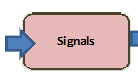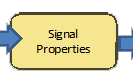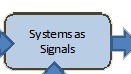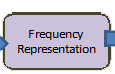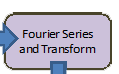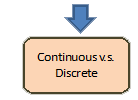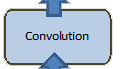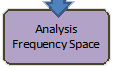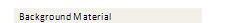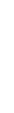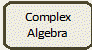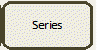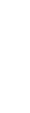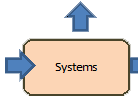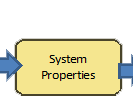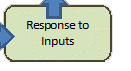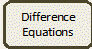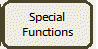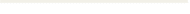Topic Online Tutorial Lecture Notes Section Notes Signals x(t) x[t] -- [ppt] [pdf] Signal Properties Even, Odd, Bounded [doc] [pdf] [ppt] [pdf] Special Signals Impulse, etc. -- [ppt] [pdf] Systems What is... -- -- System Properties LTI -- [ppt] [pdf] Response to Input Input response [doc] [pdf] [ppt] [pdf] System Responses Response [doc] [pdf] -- Convolution Convoluted Convolution [doc] [pdf] [ppt] [pdf] Systems as Signals A Signal is Can also Be a System! -- -- Frequency Representation How Frequency Can be Displayed -- -- Fourier Series and Transform Time to Frequency [doc] [pdf] [ppt] [pdf] Analysis of Frequency Space What's frequency space? -- --

 Background Material Online Tutorial Lecture Notes Section Notes Complex Algebra Exp(j π t) -- [ppt] [pdf] Series Series -- [ppt] [pdf] Difference Equations Diff-E-Q review -- -- Special Functions Foreign Functions [doc] [pdf] [ppt] [pdf]

 Useful Material Word Document Portable Document Format Section Notes Fourier Transforms [doc] [pdf] -- Fourier Transform Properties [doc] [pdf] --

Discussion Board

Discuss course material and suggestions with other students here!